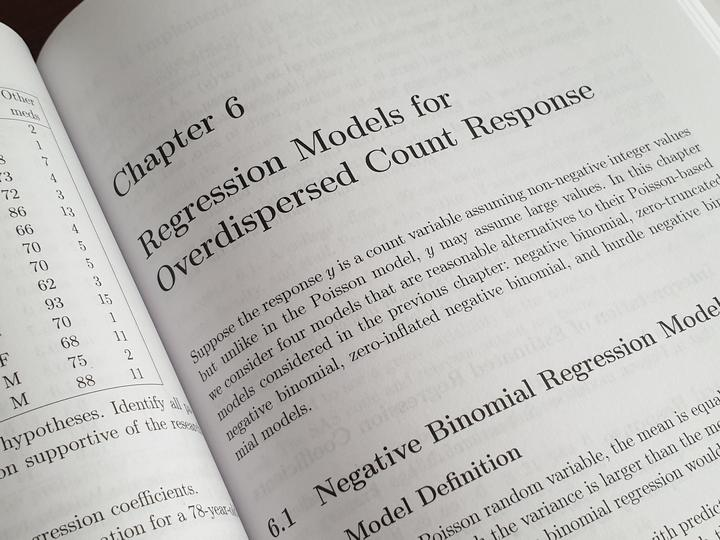# A review of Advanced Regression Models with SAS and RPhoto by Enayet Raheem on Unsplash

By Olga Korosteleva

CRC Press, 2019

Pages: xiii + 310

This book can be summarized as a cookbook of various types of regression models and their implementations with SAS and R statistical software. The chapters follow a specific pattern on the presentation starting with a brief introduction to the theory behind the technique, followed by the SAS and R implementations.

The theory is not detailed, but anyone with some mathematical statistics background will be able to review them quickly and understand them sufficiently to appreciate them as they are applied to solve practical problems.

Then a real data use case is presented and solved with SAS and R.

The SAS portion is covered with discussion while the same is implemented in R without further discussion. That is to say, anyone wanting to follow the R portion only would still be benefited to review the SAS implementations for added discussions.

At the end of each chapter, there are exercise problems that interested readers and students would find useful.

The book has a companion website with all the data in CSV format. A solution manual to the exercise problems is available for instructors.

The book starts with an introduction to the general and generalized linear regression. Then it presents the regression model for responses within the right-skewed distribution. Here the box Cox power transformation is discussed. Important regression models discussed here are the gamma model.

Then regression models for binary response are discussed in chapter 3. The binary response models discussed include logistic regression, probit regression, and complementary log log model. Particularly useful discussion includes the situations where these models can be applied. The discussions are to the point with useful details. Then the regression models for categorical responses are presented. Also presented are the regression models for count responses, including Poisson models, zero-inflated, zero-truncated, and Hurdle Poisson models, a generalized linear regression model for repeated measures data, and hierarchical regression models.

In summary, the book is a useful reference for practitioners who frequently apply regression techniques to their data. Even for seasoned statisticians, this book is convenient. It can serve as a quick reference on how to do a particular regression model along with SAS and R codes. It is especially useful for R users who will find all the various packages needed for these regression models.

Although the book covers model fitting and interpretation of estimated regression parameters, model building and variable selection are not covered due to space constraints. Also, not covered are model diagnostics.

This book is unique in the sense that it provides recipes for almost all types of regression models. The author intelligently avoided much about the theory but did not ignore them altogether. The theory is presented at a minimum level and in an amount that is necessary for those interested. However, they can be totally skipped, and someone interested only in the implementations would find this book a convenient reference.

Acknowledgements

I want to thank the CRC publisher and the International Society for Clinical Biostatistics for arranging a copy of the book for review.###### Principal Data Scientist

I support pharmaceutical companies to generate real world evidence (RWE) from real world data (RWD). The easiest way to get a response from me is to leave a comment down below. For career advice, please use email. Opinion expressed here are my own.